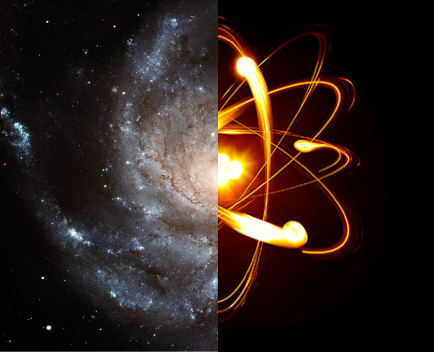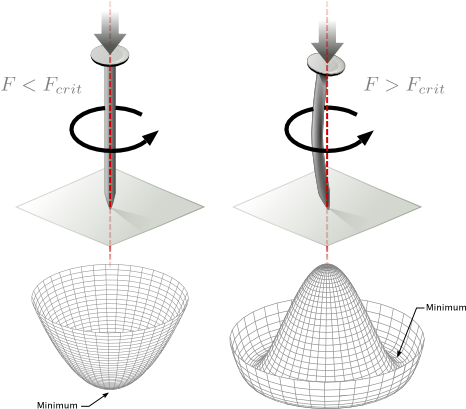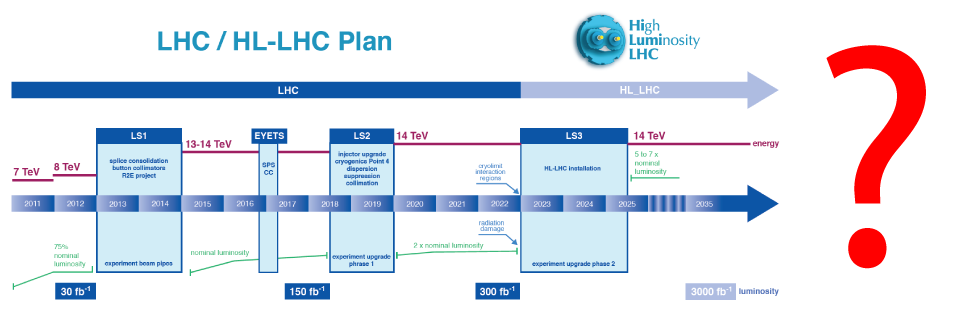1

# Physics beyond the Standard Model and the origin of the electro-weak symmetry

Many phenomena observed in Nature can be explained by our most advanced physical theories. Nevertheless these observations leave great open questions and motivate extensions of the present theories that might explain:

• From where does it come all the matter of which we are made of and surrounds us?
• What is the dark matter in the Universe?
• What drives the expansion of the Universe?

# Infinitely small, infinitely large

These questions concern aspects of the physics ruling the Universe on the largest scales, up to the size of observables Universe (and beyond?) and at the same time involve aspects of the fundamental interactions of the smallest constituents of matter. These interactions are known from direct observation up to distances as short as $$10^{-18}$$ meters (one thousandth of of the size of the proton, that is the smallest atomic nucleus) and are for the most part well described by the Standard Model of particle physics of Glashow, Weinberg, and Salam. However this model does not provide answers to the questions above because (partly or completely) lacking the necessary ingredients to formulate an answer to these question. Furthermore the model itself gives rise to further questions about the fundamental interactions, such as:

• What originates the mass of the elementary particles?

# Fundamental properties of Nature

This last question is intimately related to the above question because the elementary particles determine important properties of our Universe. For instance the size of the atoms is ruled by the Bohr radius, that is the inverse of the electron mass: $$a_0 \sim 1/m_e$$. For another instance, the masses of vector bosons and of the other elementary particles determine the times and the characteristics of the epochs of the evolution of the primordial Universe. 2 In order for elementary particles masses to exist, is necessary that the electro-weak symmetry is broken at macroscopic distances, still we know from experiments that interactions in the microscopic world respect this symmetry. Therefore a question arises, and is a main theme of my research:

• How Nature realizes the breaking of the electro-weak symmetry?

# Breaking of the electro-weak symmetry and new physics

The electro-weak interactions are the only fundamental interactions whose vector bosons, the $$W$$ and $$Z$$ bosons, are massive, hence act only on short distances, shorter than the size of an atom. In particular the electro-weak symmetry associated with the $$W$$ and $$Z$$ bosons is spontaneously broken and today we know with good accuracy that the agent of this breaking is the Higgs boson. Therefore the peculiarity of the electro-weak interactions is evident and the study of the breaking of the related symmetry is a problem of great importance in theoretical physics. Furthermore, because of the role played by this symmetry in the generation of the masses of the elementary particles, understanding the origin of the electro-weak symmetry and of the dynamics associated to its breaking is very important for the phenomenology of elementary particles and for the study of the Universe on the largest scales.

Motivated by the above considerations my research on the breaking of the electro-weak symmetry is articulated as follows:

• building new physics models for the dynamics responsible for the breaking of the electro-weak symmetry;
• studying the experimental manifestations of these models and the properties of the Standard Model that are most sensitive to new physics.

The last point is very close to the research activity of Prof. Giuseppe Degrassi. Furthermore, in some scenarios of new physics the breaking of the electro-weak symmetry can easily be linked to the dynamics responsible for the origin of neutrino masses and the pattern of masses and mixing of the Standard Model fermions that is investigated by Davide Meloni.3# New physics models

My research activity aims at building models that can explain some peculiar aspects of the Standard Model, such as for example the sensitivity of the mass of the Higgs boson to physics of very short characteristic scale (in principle shorter than what can be imagined to be probed by current and future accelerators). The models I study introduce new dynamics which explain the features of the Standard Model resolving puzzles such as that of the radiative stability of the electro-weak scale, or the origin of the masses and mixing of the fundamental particles, or provide possible candidates for the dark matter of the Universe.

These models serve the purpose of understanding the features of possible theories that extend the Standard Model and at the same time help us understand the possible experimental manifestations of new physics.# Collider physics

Particles colliders allow us to study the nature of particles interactions at very short distances. Nowadays we study interactions at the scale 1/TeV, that is to say $$10^{-18}$$ meters, where the physical laws are dictated by the Standard Model of Glashow, Salam and Weinberg. At these distances we expect to see the first signals of new physics and in particular of the dynamics responsible for the breaking of the electro-weak symmetry.

Particle colliders allows us to accelerate particles at very high energy and to observe a large number of interactions, enabling precision studies of the laws of Nature. This allows to test the predictions of the Standard Model and to observe possible new fundamental interactions. For example the recent discovery of the Higgs boson at the Large Hadron Collider has highlighted the existence of a new interactionmediated by the new particle. So far the observations indicate a good agreement with the hypothesis that this new interaction is directly proportional to the mass of the elementary particles. However, the experimental data keeps improving in precision. Therefore the sensitivity to small deviations from the above hypothesis keeps increasing making the experiments even more sensitive probes of scenarios of new physics motivated by the mystery of electro-weak symmetry breaking.